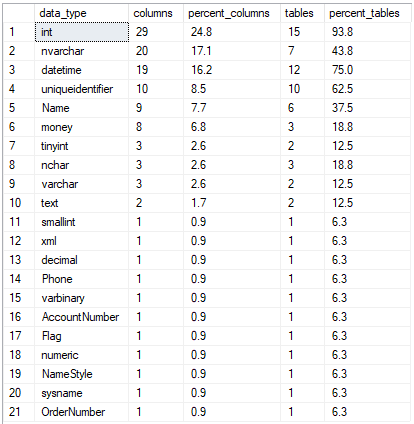## Find most used data type in Azure SQL Database

Article for:

The query below returns data types used in a database ordered by the number of their occurrence.

## Query

``````select t.name as data_type,
count(*) as [columns],
cast(100.0 * count(*) /
(select count(*) from sys.tables as tab inner join
sys.columns as col on tab.object_id = col.object_id)
as numeric(36, 1)) as percent_columns,
count(distinct tab.object_id) as [tables],
cast(100.0 * count(distinct tab.object_id) /
(select count(*) from sys.tables) as numeric(36, 1)) as percent_tables
from sys.tables as tab
inner join sys.columns as col
on tab.object_id = col.object_id
left join sys.types as t
on col.user_type_id = t.user_type_id
group by t.name
order by count(*) desc
``````

## Columns

• data_type - built-in or user data type without length or precision, e.g. int, varchar or date
• columns - number of columns in a database with this data type
• percent_columns - percentage of columns with this data type. Rows add up to 100%
• tables - number of tables in a database with this data type
• percent_tables - percentage of tables that have columns with this data type

## Rows

• One row: represents one data type
• Scope of rows: all data types used in a database
• Ordered by: number of columns in descending order

## Sample results

These results show the data types used in the AdventureWorksLT database. As you can see, the most popular data type is int - used in 29 columns representing 24.8% of all columns in 15 tables (representing 93.8% of all tables).